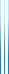# PublicationsPapers

## The carromboard problem

Yuval Filmus
Manuscript

Snepscheut came up with the following puzzle. There is a table with four coins at the center of the four sides. The goal is to get all of the coins in the same orientation, that is, all heads or all tails. You never get to see the coins (you are blindfolded), but you can turn one or two of them. Prior to each move, the table is rotated by an arbitrary, unknown multiple of 90 degrees. How many moves do you need to reach the goal?

Dĳkstra generalized the solution to $2^n$ sides. We consider an even more general problem, in which the table has $n$ sides, and the coins have $m$ “states” which are modified by addition modulo $m$ (more generally, one could think of a finite Abelian group for the state of the coins). We show that:

• The puzzle is solvable if and only if either $n=1$, $m=1$, or $n$ and $m$ are powers of the same prime.
• When m is prime, we explicitly describe all minimum-length solutions.

For similar results and more, see Rotating-table games and derivatives of words by Bar Yehuda, Etzion and Moran.